# 第一章：计算机系统结构的基本概念

## 系统结构的层次## 系统结构的分类

（1）对系统结构进行分类最常用的是Flynn分类法。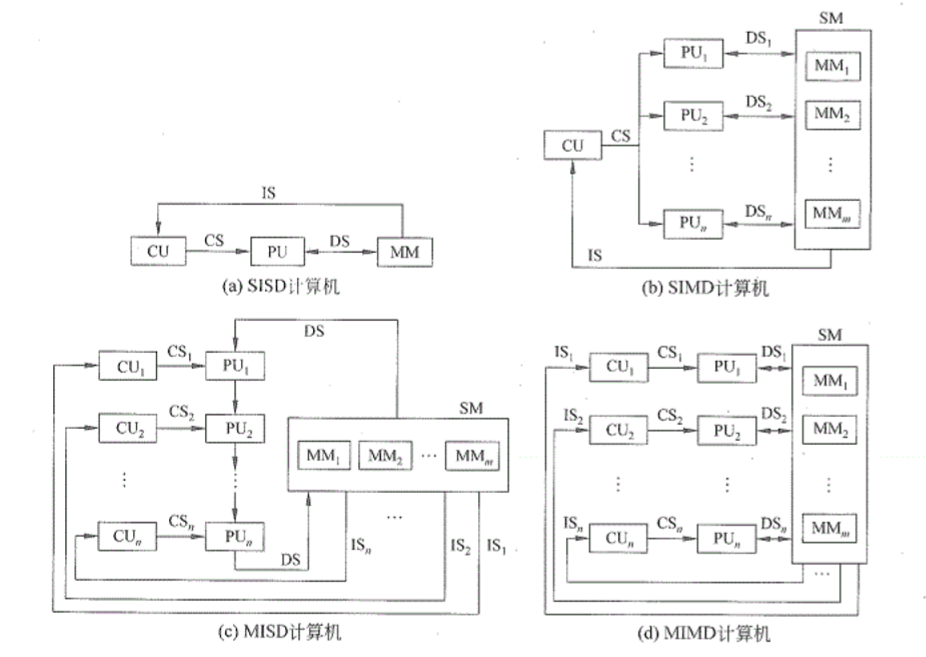（2）冯氏分类法

（3）Handler分类法

t（系统型号）＝（k，d，w）

t （系统型号）＝（k×k’，d×d’，w×w’）

## 系统结构设计的定量原理

Amdahl定律

F e = 部 件 的 执 行 时 间 总 执 行 时 间 Fe=\frac{部件的执行时间}{总执行时间}
S e = 部 件 改 进 前 耗 时 部 件 改 进 后 耗 时 Se=\frac{部件改进前耗时}{部件改进后耗时}

S = T o l d T n e w = 1 ( 1 − F e ) + F e S e S=\frac{T_{old}}{T_{new}}=\frac{1}{(1-Fe)+\frac{Fe}{Se}}

CPU性能公式

• IC：程序的指令数量
• CPI：每条指令需要的时钟周期数量

C P I = 程 序 耗 费 时 钟 周 期 数 I C CPI=\frac{程序耗费时钟周期数}{IC}
C P U 时 间 = 程 序 耗 费 时 钟 周 期 数 ∗ 时 钟 周 期 CPU时间=程序耗费时钟周期数*时钟周期

## 系统结构设计的任务## 系统结构性能测评标准

• 时间角度标准：执行时间
• 空间角度标准：吞吐率

∑ ( w i R i ) n \frac{\sum(w_iR_i)}{n}

∏ ( w i R i ) n \sqrt[n]{\prod(w_iR_i)}

## 其他概念补充# 第二章：计算机指令集结构• 指令系统结构的分类
• 寻址方式
• 指令系统的设计和优化
• 两种方向的指令系统发展
• MIPS指令系统简述

## 寻址方式

• 寄存器寻址：add r1,r2 R e g [ r 1 ] = R e g [ r 1 ] + R e g [ r 2 ] Reg[r1]=Reg[r1]+Reg[r2]
• 立即数寻址：add r1,6 R e g [ r 1 ] = R e g [ r 1 ] + 6 Reg[r1]=Reg[r1]+6
• 偏移寻址：add r1,120[r2] R e g [ r 1 ] = R e g [ r 1 ] + M e m [ 120 + R e g [ r 2 ] ] Reg[r1]=Reg[r1]+Mem[120+Reg[r2]]
• 寄存器间接寻址：add r1,[r2] R e g [ r 1 ] = R e g [ r 1 ] + M e m [ R e g [ r 2 ] ] Reg[r1]=Reg[r1]+Mem[Reg[r2]]
• 直接寻址：add r1,(1000) R e g [ r 1 ] = R e g [ r 1 ] + M e m [ 1000 ] Reg[r1]=Reg[r1]+Mem
• 存储器间接寻址：add r1,@[r2] R e g [ r 1 ] = R e g [ r 1 ] + M e m [ M e m [ R e g [ r 2 ] ] ] Reg[r1]=Reg[r1]+Mem[Mem[Reg[r2]]]

## 指令系统的设计和优化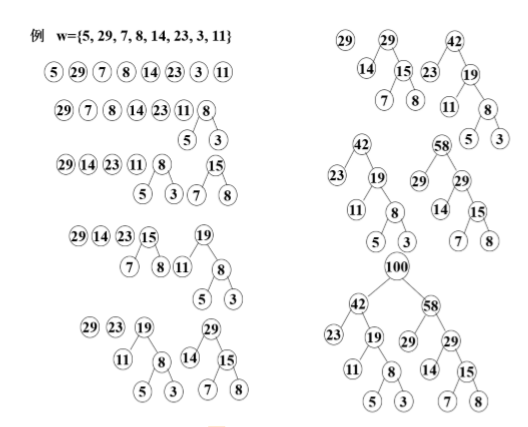• 信息熵的计算（最短平均码长）：
H = − ∑ i n p i ∗ l o g 2 ( p i ) H=-\sum^n_{i}pi*log_2(pi)
• 平均码长的计算：
L = ∑ i n p i ∗ l i L=\sum^n_ipi*li
• 信息冗余度（无用信息的比例）的计算：
r = L − H L r=\frac{L-H}{L}
需要注意的是平均码长和最短平均码长不是一种东西！，信息熵的是用来表示某个信息能带来多少信息量，通俗说就是度量信息量的有多少计算。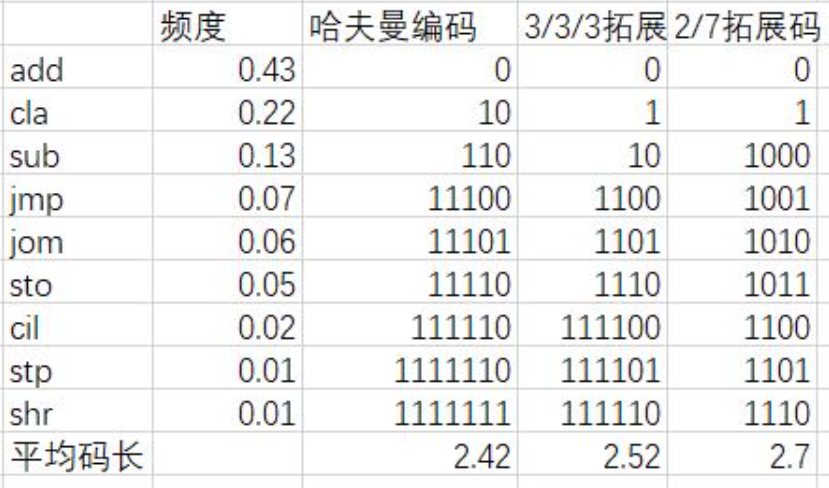n1/n2/n3编码到底怎样编出来的呢？2/7编码：15/15/15编码：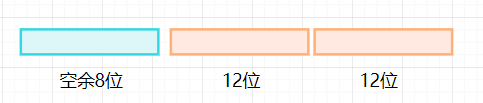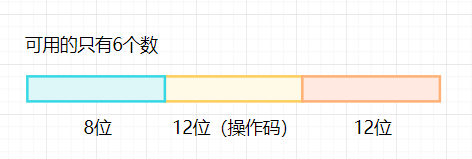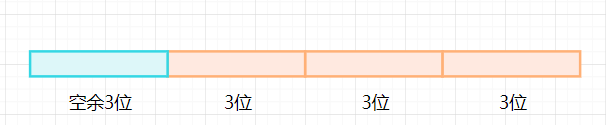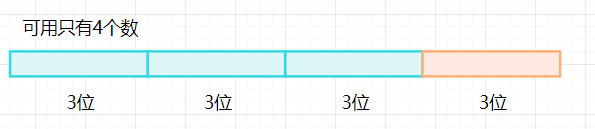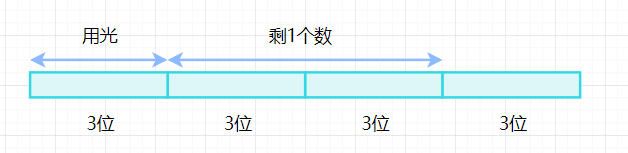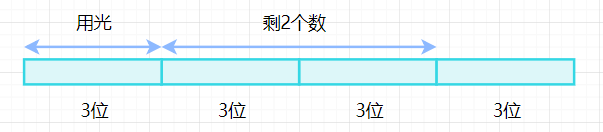## 两种方向的指令系统发展

CISC方向

RISC方向

➢ 采用简单而又统一的指令格式，并减少寻址方式； 指令字长都为32位或64位；
➢ 指令的执行在单个机器周期内完成； (采用流水线机制)
➢ 大多数指令都采用硬连逻辑来实现；

02-164万+
01-081万+08-161246
09-151万+
01-041145
04-05291
06-3090
06-24304
06-111133
11-183987